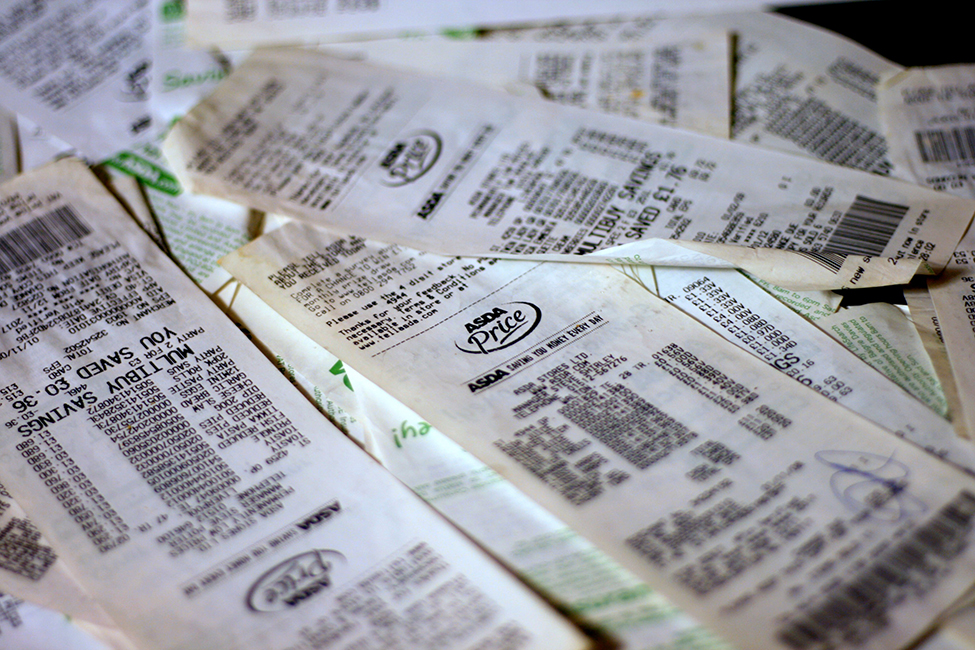## Introduction to The Chi-Square DistributionThe chi-square distribution can be used to find relationships between two things, like grocery prices at different stores. (credit: Pete/flickr)
Have you ever wondered if lottery numbers were evenly distributed or if some numbers occurred with a greater frequency? How about if the types of movies people preferred were different across different age groups? What about if a coffee machine was dispensing approximately the same amount of coffee each time? You could answer these questions by conducting a hypothesis test.

You will now study a new distribution, one that is used to determine the answers to such questions. This distribution is called the chi-square distribution.

In this chapter, you will learn the three major applications of the chi-square distribution:

the goodness-of-fit test, which determines if data fit a particular distribution, such as in the lottery example
the test of independence, which determines if events are independent, such as in the movie example
the test of a single variance, which tests variability, such as in the coffee example

### NOTE

Though the chi-square distribution depends on calculators or computers for most of the calculations, there is a table available. TI-83+ and TI-84 calculator instructions are included in the text.

### Activity

Look in the sports section of a newspaper or on the Internet for some sports data (baseball averages, basketball scores, golf tournament scores, football odds, swimming times, and the like). Plot a histogram and a boxplot using your data. See if you can determine a probability distribution that your data fits. Have a discussion with the class about your choice.